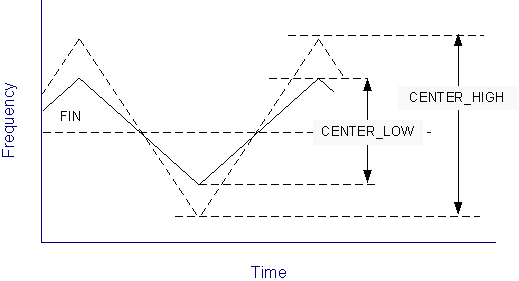# AR# 51561

## 説明fig1.pngfig2.png

When using spread spectrum in SS_MODE(CENTER_HIGH) or SS_MODE( CENTER_LOW), the center spread will increase the effective frequency ranges.
No versions of ISE Design Suite support automatic timing analysis for CENTER_HIGH or CENTER_LOW. Current versions Vivado Design Suite also do not support automatic timing analysis for CENTER_HIGH or CENTER_LOW.

## ソリューション

スペクトラム拡散の周波数範囲を考慮するため、入力クロックに手動で制約を設定する必要があります。

 入力周波数 [MHz] M 入力周波数調整 (FIN_SS) SS_MODE (CENTER_HIGH) 25 < FIN < 35 M=28 FIN_SS = FIN * 56 / 55 35 < FIN < 50 M=21 FIN_SS = FIN * 42 / 41 M=22 FIN_SS = FIN * 44 / 43 50 < FIN < 75 M=28 FIN_SS = FIN * 56 / 55 75 < FIN < 150 M=21 FIN_SS = FIN * 42 / 41 M=22 FIN_SS = FIN * 44 / 43 SS_MODE (CENTER_LOW) 25 < FIN < 35 M=56 FIN_SS = FIN * 112 / 111 35 < FIN < 50 M=42 FIN_SS = FIN * 84 / 83 M=44 FIN_SS = FIN * 88 / 87 50 < FIN < 75 M=56 FIN_SS = FIN * 112 / 111 75 < FIN < 150 M=42 FIN_SS = FIN * 84 / 83 M=44 FIN_SS = FIN * 88 / 87 SS_MODE (DOWN_HIGH) 25 < FIN < 35 M=28 FIN_SS = FIN 35 < FIN < 50 M=21、22 FIN_SS = FIN 50 < FIN < 75 M=28 FIN_SS = FIN 75 < FIN < 100 M=21、22 FIN_SS = FIN 100 < FIN < 150 M=21、22 FIN_SS = FIN SS_MODE (DOWN_LOW) 25 < FIN < 35 M=56 FIN_SS = FIN 35 < FIN < 50 M=42、44 FIN_SS = FIN 50 < FIN < 75 M=56 FIN_SS = FIN 75 < FIN < 100 M=42、44 FIN_SS = FIN 100 < FIN < 150 M=42、44 FIN_SS = FIN

25MHz の入力クロックの場合のタイミング制約

SS_MODE (CENTER_HIGH) = 25 * 56/55 = 25.45MHz

SS_MODE (CENTER_LOW) = 25 * 112/111 = 25.23MHz

SS_MODE (DOWN_HIGH) および SS_MODE (DOWN_LOW) では拡散により周波数が低下するので、入力クロックに変更は不要です。

80MHz のクロックで (CENTER_HIGH) では M = 22、(CENTER_LOW) では M = 44

SS_MODE(CENTER_HIGH) =80 *44/43 = 81.86MHz

SS_MODE (CENTER_LOW) =80*88/87 = 80.92MHz

SS_MODE (DOWN_HIGH) および SS_MODE (DOWN_LOW) では拡散により周波数が低下するので、入力クロックに変更は不要です。